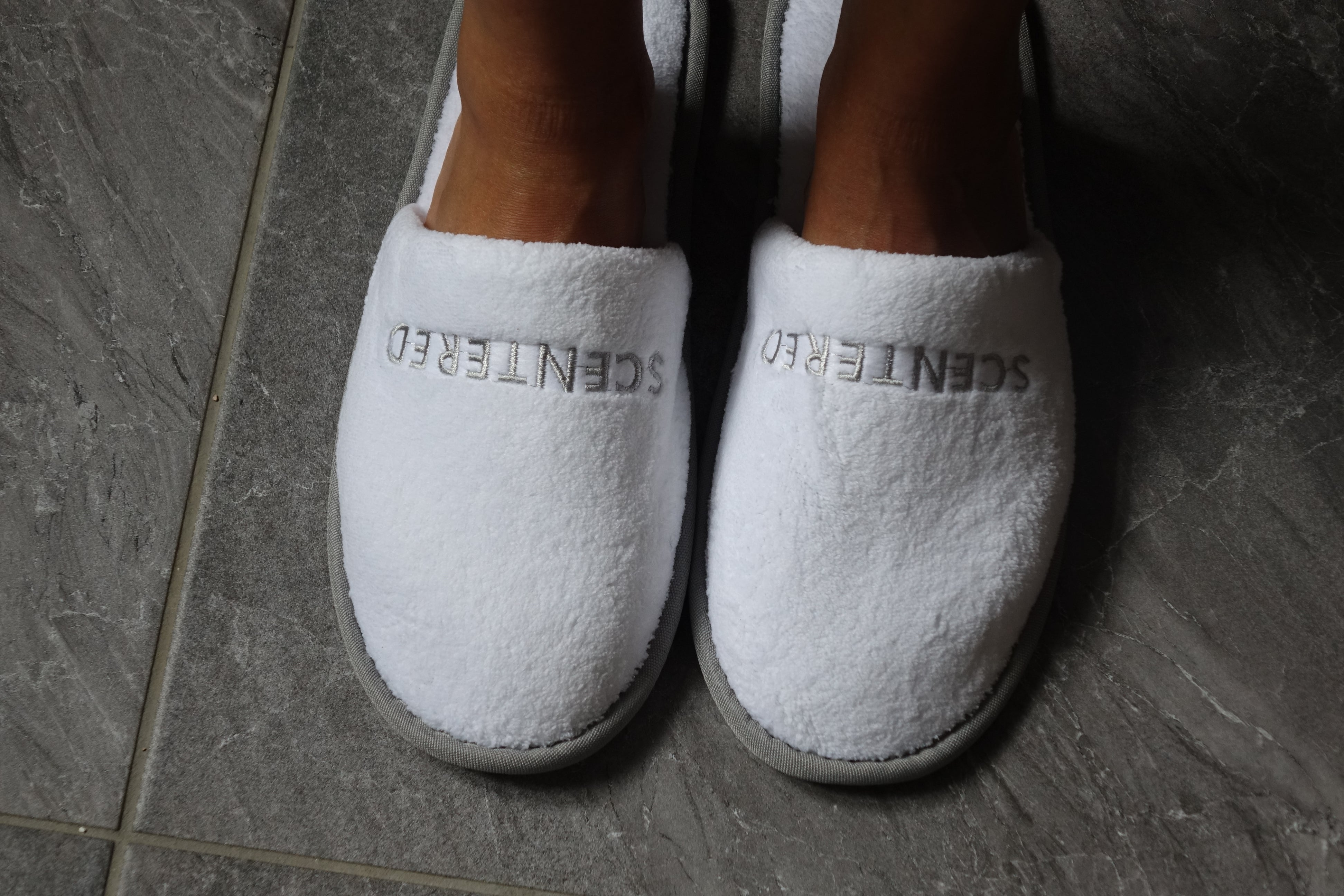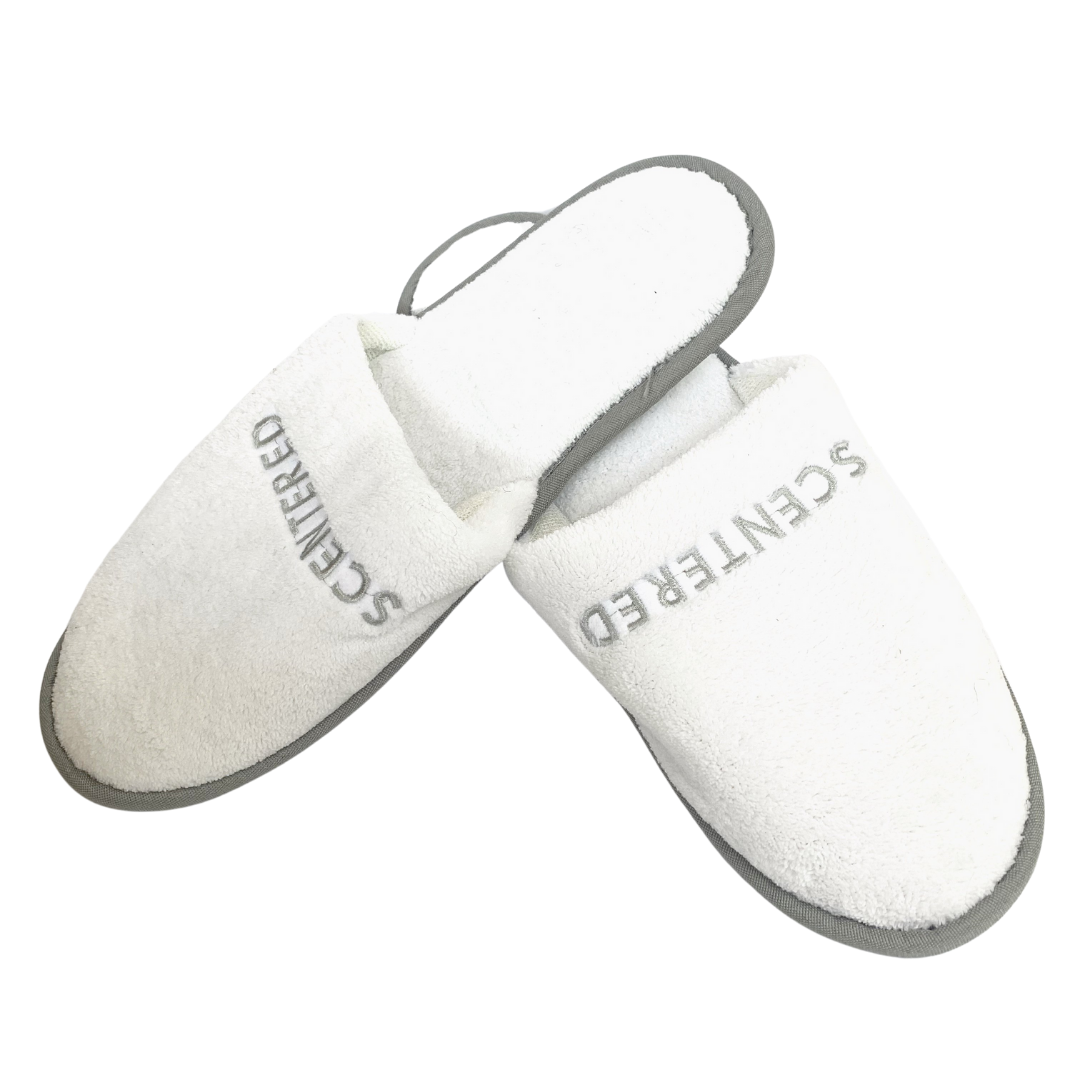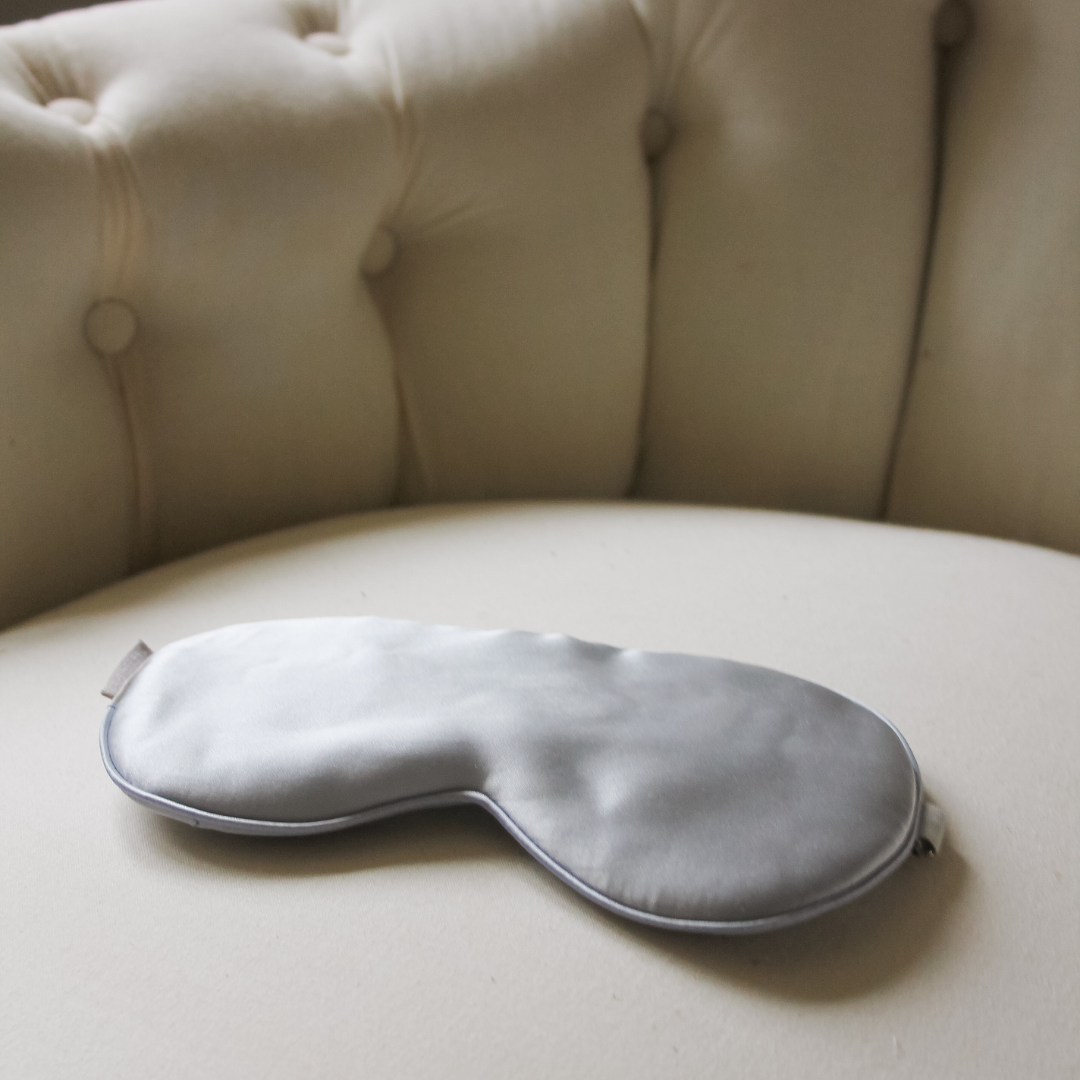### H\$A\$P\$P\$Y\$ T\$r\$a\$v\$e\$l\$ A\$r\$o\$m\$

£16.95### S\$l\$i\$p\$

£6.00### S\$i\$l\$k\$ E\$y\$

£19.99### L\$a\$r\$g\$e\$ P\$o\$u\$c\$h

£9.99### D\$E\$-S\$T\$R\$E\$S\$S\$ B\$o\$d\$y\$ a

£16.00### D\$E\$-S\$T\$R\$E\$S\$S\$

£17.00### D\$E\$-S\$T\$R\$E\$S\$S\$ C\$o

£17.00### D\$E\$-S\$T\$R\$E\$S\$S\$ W\$e\$l\$l\$b\$e\$i\$n\$g\$ R\$i\$t\$u

De £16.00### S\$L\$E\$E\$P\$ W\$E\$L\$L\$ W\$e\$l\$l\$b\$e\$i\$n\$g\$ R\$i\$t\$u\$a\$l\$ A\$r\$o\$

£17.90### E\$S\$C\$A\$P\$E\$ W\$e\$l\$l\$b\$e\$i\$n\$g\$ R\$i\$t\$u\$a

De £16.90### S\$L\$E\$E\$P\$ W\$E\$L\$L\$ W\$e\$l\$l\$b\$e\$i\$n\$g\$ R\$i\$t\$

De £16.00### H\$A\$P\$P\$Y\$ W\$e\$l\$l\$b\$e\$i\$n\$g\$ R\$i\$t\$u\$a\$

De £16.90### L\$O\$V\$E\$ W\$e\$l\$l\$b\$e\$i\$n\$g\$ R\$i\$t\$u\$a\$l

De £16.90### F\$O\$C\$U\$S\$ W\$e\$l\$l\$b\$e\$i\$n\$g\$ R\$i\$t\$u\$a\$

De £16.90### C\$o\$t\$t\$o\$n\$ P\$y\$j\$

£2.95£9.99